# Calculating Partial Q Values for Photonic Crystal Slab Using the Q-Finder Utility

The RSoft Photonic Device Tool, FullWAVE, is an FDTD-based simulation tool and includes the Q-Finder utility to automate the calculation of the central frequency, quality factor Q, and spatial profiles of resonant modes.

Q-Finder utilizes several methods to calculate Q factors, including a Fast Harmonic Analysis technique and an energy decay method, both described in Section 8.A.2 of the FullWAVE manual. The FHA method is always used and measures the Q-Factor of the entire mode, whereas the energy decay method takes more time but can be used to measure partial-Qs for in-plane and out-of-plane losses for photonic crystal slabs.

Optical micro-cavities with high Q factors are significant for both lightwave technology and research in quantum optics. They can be used to create low threshold micro-lasers, nonlinear optical elements, narrow linewidth, and wavelength selective filters. Planar 2D Photonic Crystal (PhC) slab waveguides with localized defects offer an appealing architecture for such work.

One of the biggest challenges is the construction of optical micro-cavities with small mode volumes and large quality factors for efficient localization of light. For PhC slabs, the total Q factor can be separated into in-plane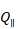and out-of-plane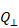: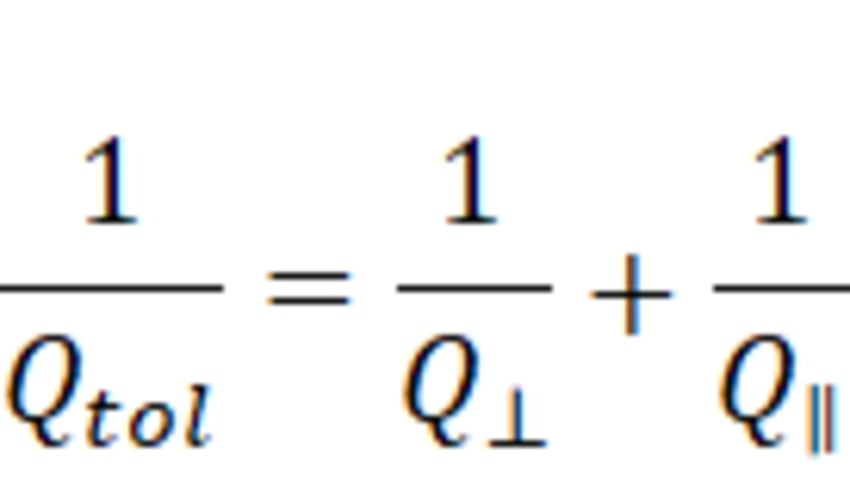In this article, we demonstrate how to use the Q-Finder utility to calculate partial Q values for a PhC slab micro-cavity. The structure has been drawn in the RSoft CAD as shown in Figure 1. It consists of a silicon slab with a hexagonal array of air holes. The slab thickness is 0.75*Period and the hole radius is 0.3*Period. The central defect has a radius of 0.45*Period. The design contains monitors that will measure the total energy density (dark blue), and the power through the six exit faces (yellow) which will be used to calculate the partial Q. Assuming that the cavity mode is well inside the photonic band gap, this gives the upper limit for the total Q factor of the cavity mode.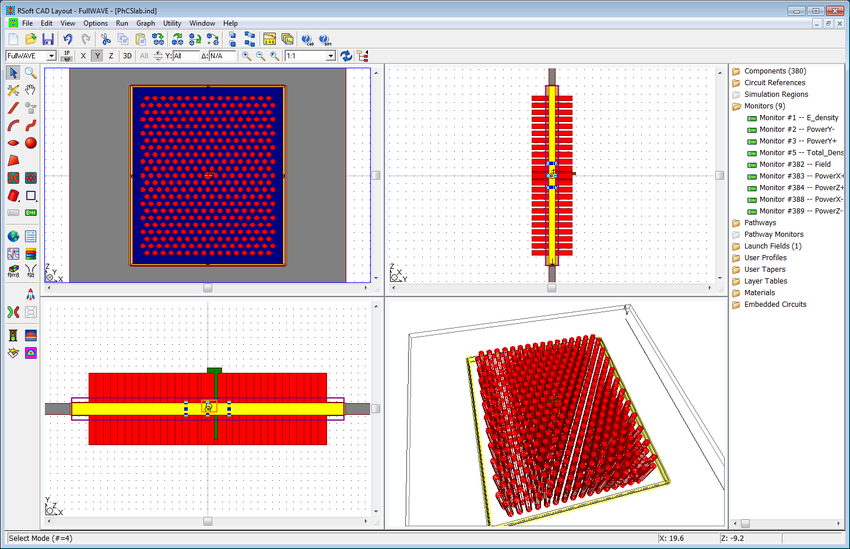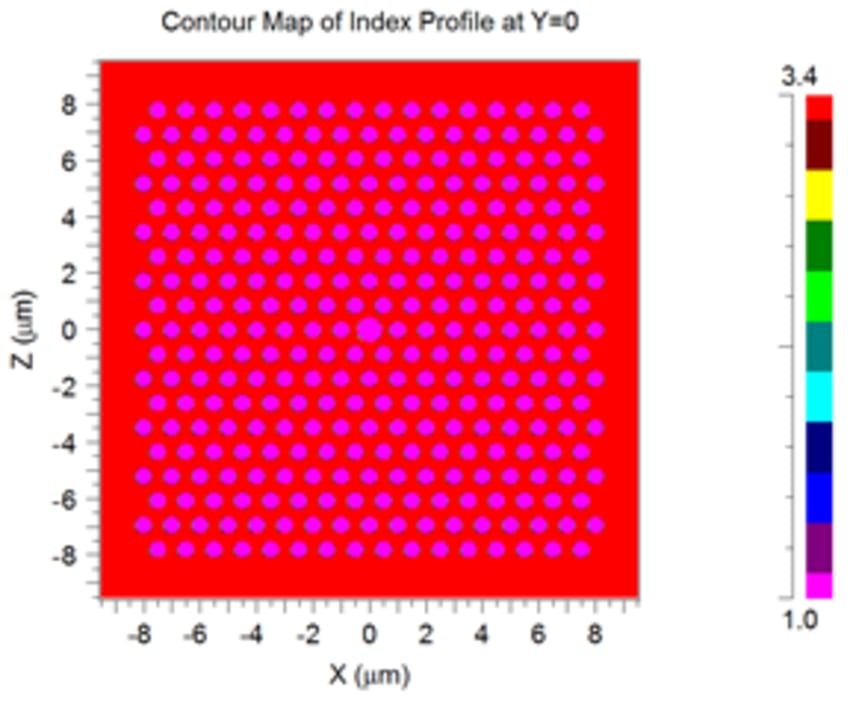Figure 1: PhC slab structure in the RSoft CAD (left) and index profile in xz plane (right)

The basic simulation settings such as grid size, domain, boundary conditions, etc., are controlled by FullWAVE. The file is configured to study the effect of the grid size on the result (which is always recommended!). We have created a symbol Nper which sets the number of grid points per period. Other parameters related to Q calculation are set in the Q-Finder configuration dialog shown in Figure 2. Here we choose to search for modes near a frequency of 0.25 using a relatively large tolerance. Q-Finder uses a series of successively longer simulations (in number of time steps) to find and refine the cavity mode. For this calculation, we will use four simulations, each with 2^N time steps where N is 13, 13, 16, and 16. It is worth noting that since the time step is a function of the grid size, reducing the grid size via Nper would effectively reduce the spectral resolution of the calculation. To avoid this, we created and added a factor (TPowOff) to each time length so that the simulation stop time (and therefore spectral resolution) is invariant to grid size changes.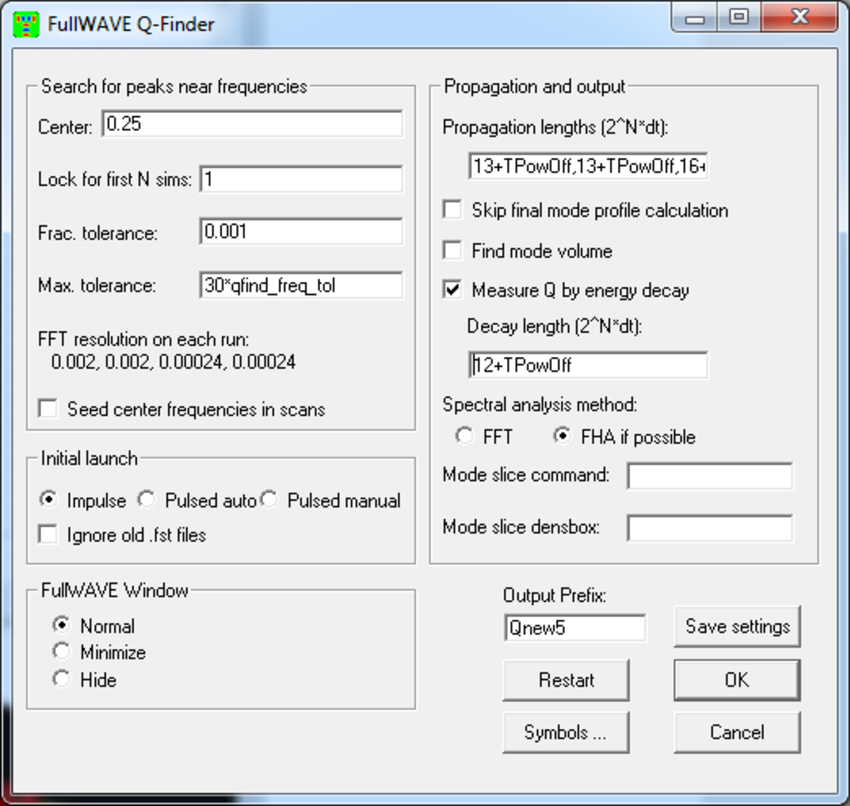Figure 2: Q-Finder simulation dialog for this structure.

Please note the following requirements to calculate partial Q values:

1. One total density monitor to cover the mode spatial range you are interested in for the energy decay calculation.
2. One field time monitor for the FHA Q calculation.
3. Multiple power monitors positioned at the sides of interest of the total density monitor for the partial Q calculation in that direction.
4. Selection of the energy decay option in Q-Finder.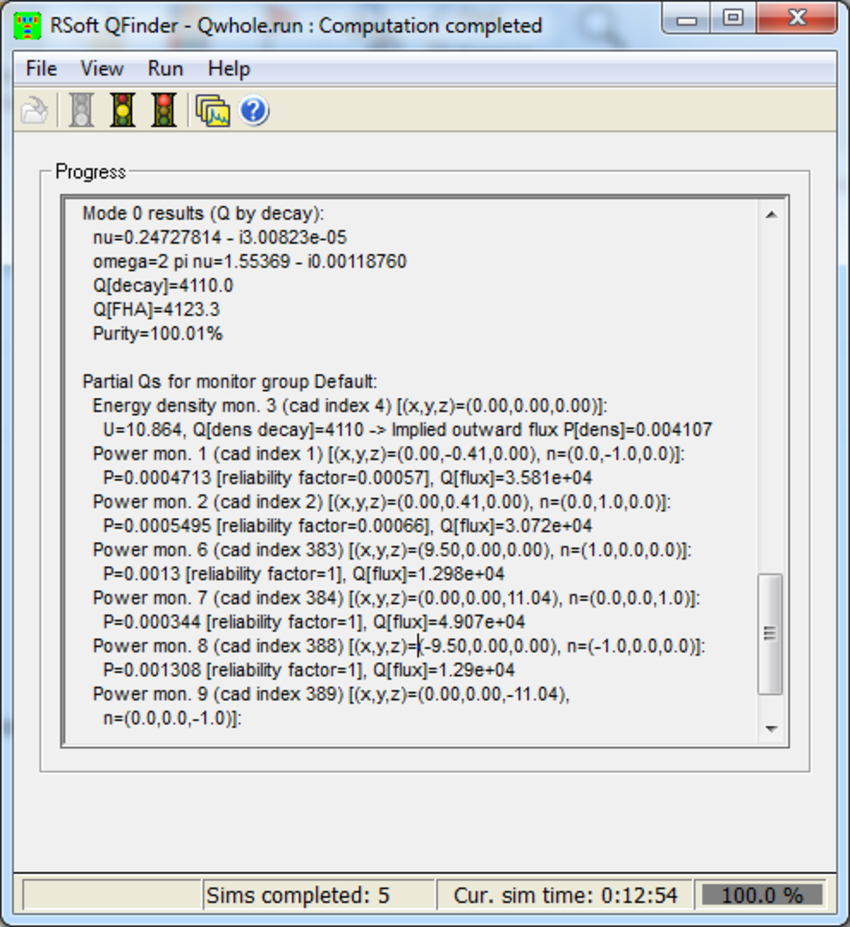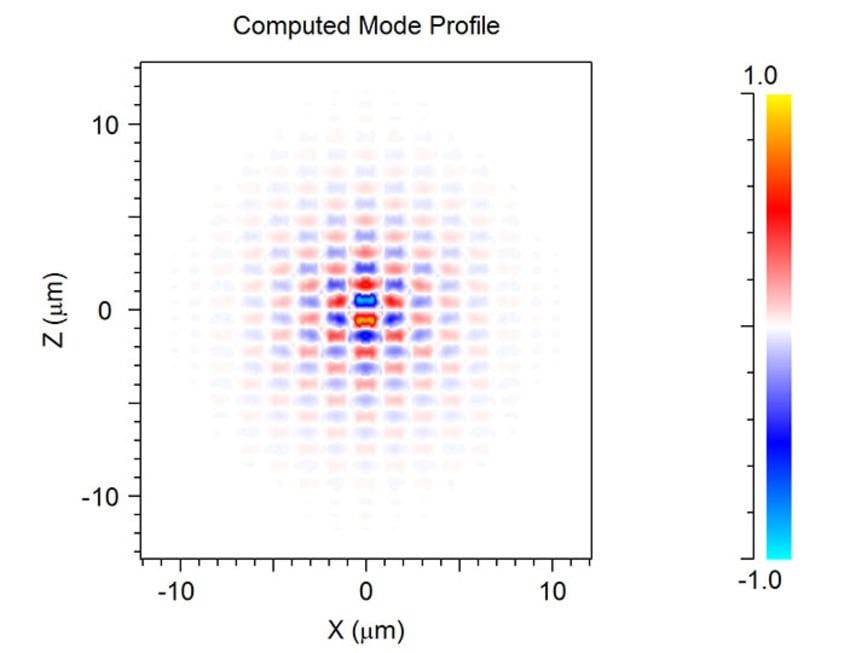Figure 3: Q-Finder simulation progress window (left) and the cavity mode found (right)

Once started, the simulation progress can be tracked in the Q-Finder window. Upon completion, the total Q and partial Q values will be reported. The mode profile shown in Figure 3 can be opened by DataBROWSER. Using 8 grid points per period (Nper=8), we obtained total Q of about 4000, in-plane of about 5130, and out-of-plane  of about 17900.  Higher accuracy would require a smaller grid size (higher Nper).

The calculation of a 3D cavity mode is numerically intensive, and so it is worth noting that we used symmetry boundary conditions which, if there is full symmetry, results in an 8x problem size reduction. It is very important to select the symmetry conditions carefully, since some field components may be symmetric while others are anti-symmetric within the same mode. See Section 6.C.3 of the FullWAVE manual for more information, including a table to use the desired symmetry.

Please contact the RSoft technical support team at photonics_support@synopsys.com for more information.

Reference:

1   K. Srinivasan and O. Painter, “Fourier space design of high-Q cavities in standard and compressed hexagonal lattice photonic crystals,” Optics Express 11, 579 (2003).